### Home > CALC > Chapter Ch2 > Lesson 2.3.3 > Problem2-126

2-126.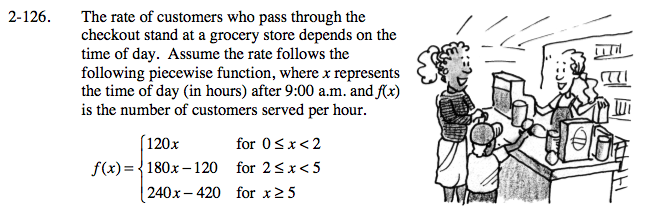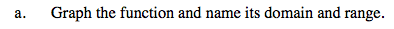Your sketch should have three linear pieces.

The domain is given by the piecewise function above.

The range can be seen on the graph. What is the highest and lowest y-value? Are there any holes or jumps on that interval?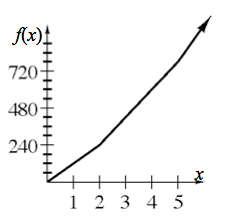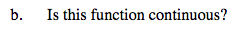We know that the graph has a starting point (0,0) so, it could only be continuous when x > 0.

Be mindful of the 3 conditions of continuity: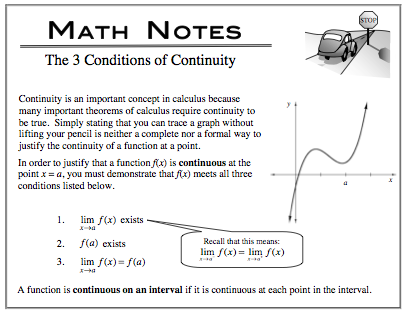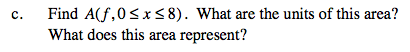Area = k(base)(height), where k is some constant. The base is determined by the units on the x-axis. The height is determined by the units on the y-axis. Multiply.